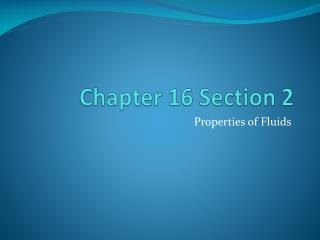# Chapter 16 Section 2 - PowerPoint PPT PresentationDownload PresentationChapter 16 Section 2

Chapter 16 Section 2Download Presentation## Chapter 16 Section 2

- - - - - - - - - - - - - - - - - - - - - - - - - - - E N D - - - - - - - - - - - - - - - - - - - - - - - - - - -
##### Presentation Transcript

1. Chapter 16 Section 2 Properties of Fluids

2. Buoyancy • Fluid = a liquid OR gas • Buoyancy = The ability of a fluid (a liquid or a gas) to exert an upward force on an object immersed in the fluid • If the object’s weight is equal to the buoyant force, the object will float • If the object’s weight is more than the buoyant force, the object will sink

3. Archimedes’ Principle • The buoyant force on an object is equal to the weight of the fluid displaced by the object • If you place an object in water, it will push water out of the way as it sinks • If the weight of the water displaced = the block’s weight, it floats • If the weight of the water displaced < the block’s weight, it sinks

4. Density • Mass per unit volume (g/L) • Wood and steel blocks example • An object will float if its density is less than the density of the fluid it is placed in • How does a steel boat float? • Density and buoyancy experiment

5. Pascal’s Principle • Pressure = force exerted per unit area • P = F/A • Pressure applied to a fluid is transmitted throughout the fluid (pressure remains constant throughout a fluid) • If P1=P2, then F1/A1 = F2/A2

6. Pressure-Force Equation • Page 487 • A hydraulic lift is used to lift a heavy machine that is pushing down on a 2.3 meter sq. piston (A1) with a force (F1) of 3700 N. What force (F2) needs to be exerted on a 0.072 meter sq. piston (A2) to lift the machine?

7. Pressure-Force Equation • Your formula • P1 = P2 • F1/A1 = F2/A2 • 3700 N = F2 . 2.8 m sq. 0.072 m sq. • F2=95 N • A heavy crate applied a force of 1500 N on a 25 m sq. piston. What force need to be exerted on the 0.80 m sq. piston to lift the crate?

8. Bernoulli’s Principle • As the velocity of a fluid increases, the pressure exerted by the fluid decreases • Blowing on paper • Hose end sprayer

9. Fluid Flow • Viscosity = Resistance to flow by a fluid • Water = low viscosity (flows easily) • Cold syrup = high viscosity (flows slowly)